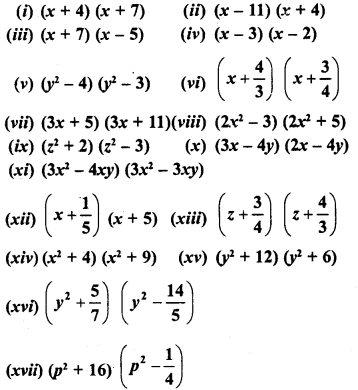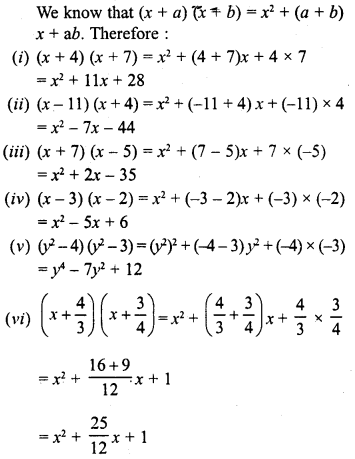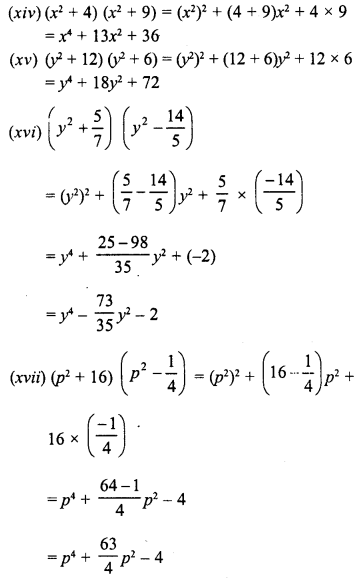## RD Sharma Class 8 Solutions Chapter 6 Algebraic Expressions and Identities Ex 6.7

These Solutions are part of RD Sharma Class 8 Solutions. Here we have given RD Sharma Class 8 Solutions Chapter 6 Algebraic Expressions and Identities Ex 6.7

Other Exercises

Question 1.
Find the following products :Solution:Question 2.
Evaluate the following :
(i) 102 x 106
(ii) 109 x 107
(iii) 35 x 37
(iv) 53 x 55
(v) 103 x 96
(vi) 34 x 36
(vii) 994 x 1006
Solution:
(i) 102 x 106 = (100 + 2) (100 + 6)
= (100)2 + (2 + 6) x 100 + 2 x 6
= 10000 + 800 + 12 = 10812

(ii) 109 x 107 = (100 + 9) (100 + 7)
= (100)2 + (9 + 7) x 100 + 9 x 7
=10000 + 1600 + 63 = 11663

(iii) 35 x 37 = (30 + 5) (30 + 7)
= (30)2 + (5 + 7) x 30 + 5 x 7
= 900 + 12 x 30 + 35
= 900 + 360 + 35 = 1295

(iv) 53 x 55 = (50 + 3) (50 + 5)
= (50)2 + (3 + 5) x 50 + 3 x 5
= 2500 + 8 x 50 + 15
= 2500 + 400+ 15 = 2915

(v)103 x 96 = (100 + 3) (100-4)
= (100)2 + (3 – 4) x 100 + 3 x (-4)
= 10000+ (-1) x 100-12
= 10000 – 100 – 12 = 10000 – 112 = 9888

(vi) 34 x 36 = (30 + 4) (30 + 6)
= (30)2 + (4 + 6) x 30 + 4 x 6
= 900 + 10 x 30 + 24
= 900 + 300 + 24 = 1224

(vii) 994 x 1006 = (1000 – 6) (1000 + 6)
= (1000)2 + (-6 + 6) x 1000 + (-6) x 6
= 1000000 + 0-36
= 1000000-36 = 999964

Hope given RD Sharma Class 8 Solutions Chapter 6 Algebraic Expressions and Identities Ex 6.7 are helpful to complete your math homework.

If you have any doubts, please comment below. Learn Insta try to provide online math tutoring for you.